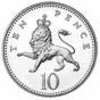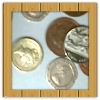#### You may also like### Coin Tossing Games

You and I play a game involving successive throws of a fair coin. Suppose I pick HH and you pick TH. The coin is thrown repeatedly until we see either two heads in a row (I win) or a tail followed by a head (you win). What is the probability that you win?### Win or Lose?

A gambler bets half the money in his pocket on the toss of a coin, winning an equal amount for a head and losing his money if the result is a tail. After 2n plays he has won exactly n times. Has he more money than he started with?A counter is placed in the bottom right hand corner of a grid. You toss a coin and move the star according to the following rules: ... What is the probability that you end up in the top left-hand corner of the grid?

# Who's the Winner?

### Why do this problem?

This problem offers the opportunity to use probability in an authentic sporting context. It introduces the idea of using a probability model to make predictions, and then to refine the model using real data.

### Possible approach

Introduce the idea of two equally matched teams (teams who are equally likely to score the next goal).

"If two goals are scored in a match, what different results are possible?"
"What are the probabilities of the different results?"

Give students time to work out the probabilities. While they are working, circulate and see what methods are being used.

Bring the class together and share different approaches (this may include approaches based on the incorrect assumption that win, loss and draw are equally likely).

With some classes, it may be appropriate to simulate the matches using dice. For example, the even numbers could correspond to one team scoring and the odd numbers could correspond to the other team scoring.

Next, introduce Charlie's and Alison's models, and allow some time for students to discuss their "gut feelings" about which might be more accurate, based on their own experiences.
Then allow time for students to simulate the matches using dice again, or to work out the probabilities using one of the methods discussed earlier (perhaps drawing attention to the efficiency of tree diagrams).

Students may be curious to know how accurately the models reflect reality; the possible extension below suggests how this can be explored.

### Key questions

Why are the results "win", "draw" and "lose" not all equally likely?

Is the second goal independent of the first goal?
What data could be collected to evaluate the models?

### Possible support

Ask pairs of students to simulate the different models using dice, and collect together all the results. Then introduce tree diagrams to explain the results of the simulation.

### Possible extension

Students could find data (perhaps from school or local teams) and decide on criteria for identifying "closely matched" teams. Then matches between closely matched teams where exactly two goals were scored can be analysed to see which model best fits the data.

Students could refine the models based on the data, and could critique the models and the assumptions made in evaluating them.